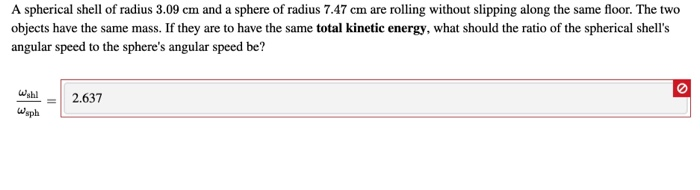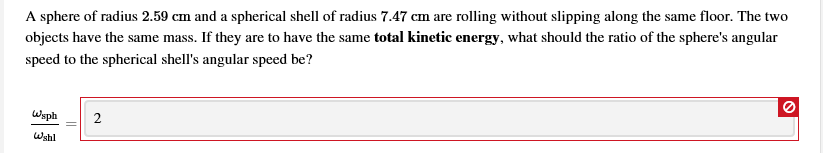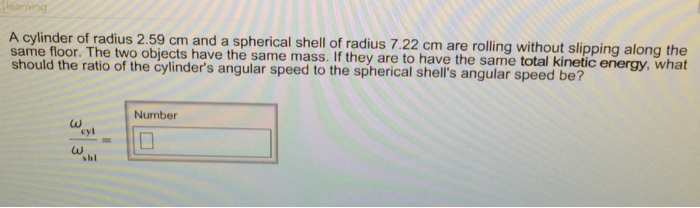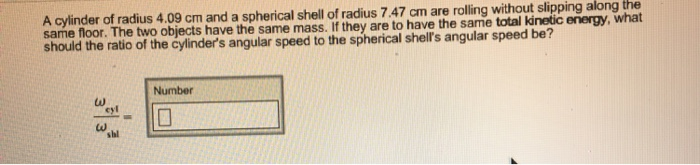Question
A sphere of radius 1.34 cm and a spherical shell of radius 7.72 cm are rolling without slipping along the same floor. The two objects have the same mass. If they areto have the same total kinetic energy, what should the ratio of the sphere's angular speed to the spherical shell's angular speed be?

We need at least 10 more requests to produce the answer.

0 / 10 have requested this problem solution

The more requests, the faster the answer.

All students who have requested the answer will be notified once they are available.

#### Earn Coins

Coins can be redeemed for fabulous gifts.

Similar Homework Help Questions
• ### A spherical shell of radius 3.09 cm and a sphere of radius 7.47 cm are rolling...A spherical shell of radius 3.09 cm and a sphere of radius 7.47 cm are rolling without slipping along the same floor. The two objects have the same mass. If they are to have the same total kinetic energy, what should the ratio of the spherical shell's angular speed to the sphere's angular speed be? Will Wsph 2.637

• ### A spherical shell of radius 3.09 cm and a sphere of radius 7.47 cm are rolling...A spherical shell of radius 3.09 cm and a sphere of radius 7.47 cm are rolling without slipping along the same floor. The two objects have the same mass. If they are to have the same total kinetic energy, what should the ratio of the spherical shell's angular speed to the sphere's angular speed be? Wahl 2.637 wsph

• ### What should the ratio be?

A spherical shell of radius 2.34 cm and a cylinder of radius 6.97 cm are rolling without slipping along the same floor. The two objects have the same mass. If they areto have the same total kinetic energy, what should the ratio of the spherical shell's angular speed to the cylinder's angular speed be?

• ### Please solve this question, and show steps. Thank you so much. A sphere of radius 2.59...Please solve this question, and show steps. Thank you so much. A sphere of radius 2.59 cm and a spherical shell of radius 7.47 cm are rolling without slipping along the same floor. The two objects have the same mass. If they are to have the same total kinetic energy, what should the ratio of the sphere's angular speed to the spherical shell's angular speed be? Wsph Wsph = 2 shil الها

• ### A cylinder of radius 2.59 cm and a spherical shell of radius 7.22 cm are rolling...A cylinder of radius 2.59 cm and a spherical shell of radius 7.22 cm are rolling without slipping along the same floor. The two objects have the same mass. If they are to have the same total kinetic energy, what should the ratio of the cylinder's angular speed to the spherical shell's angular speed be? Omega_cyl = omega_shl =

• ### A spherical shell of radius 1.34 cm and a cylinder of radius 8.22 cm are rolling...A spherical shell of radius 1.34 cm and a cylinder of radius 8.22 cm are rolling without slipping along the same floor. The two objects have the same mass. If they are to have the same total kinetic energy, what should the ratio of the spherical shell\'s angular speed to the cylinder\'s angular speed be?

• ### Show all work and provide the calculation A cylinder of radius 4.09 cm and a spherical...Show all work and provide the calculation A cylinder of radius 4.09 cm and a spherical shell of radius 7.47 cm are rolling without slipping along the same floor. The two objects have the same mass. If they are to have the same total kinetic energy, what should the ratio of the cylinders angular speed to the spherical shell's angular speed be? Number cyl sal

• ### A cylinder of radius 3.09cm and a spherical shell of radius 7.22cm are rolling without slipping...

A cylinder of radius 3.09cm and a spherical shell of radius 7.22cm are rolling without slipping along the same floor. The two objects have the same mass. If they are to have the same total KE, what should the ratio of the cylinders angular speed to the spherical shell's speed be?

• ### A cylinder of radius 3.09 cm3.09 cm and a sphere of radius 8.47 cm8.47 cm are...

A cylinder of radius 3.09 cm3.09 cm and a sphere of radius 8.47 cm8.47 cm are rolling without slipping along the same floor. The two objects have the same mass. If they are to have the same total kinetic energy, what should the ratio of the cylinder's angular speed to the sphere's angular speed be? ?cyl?sph=